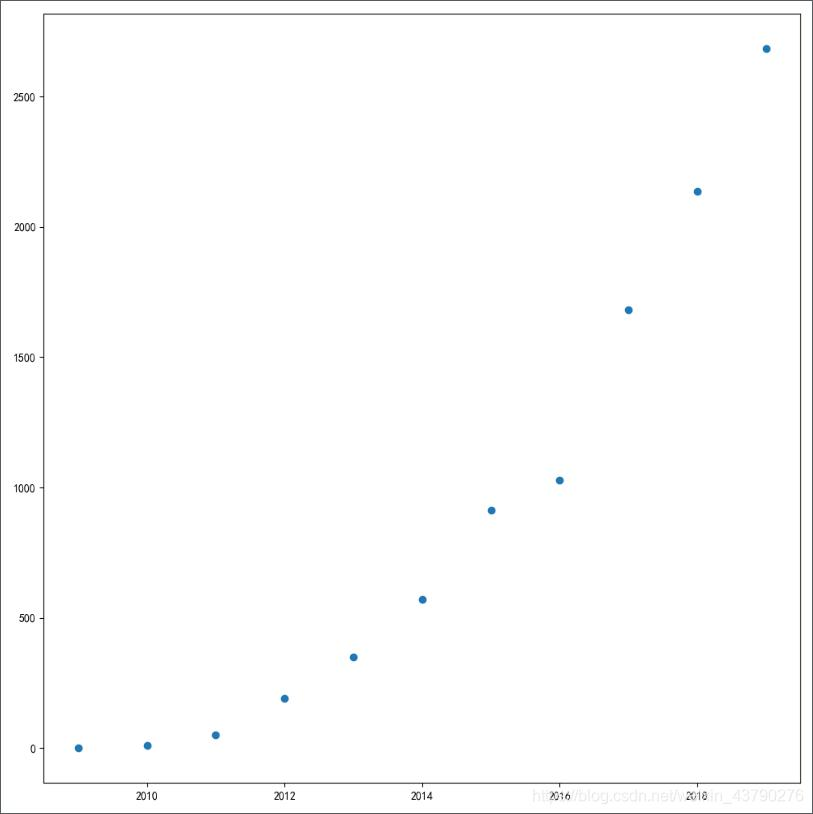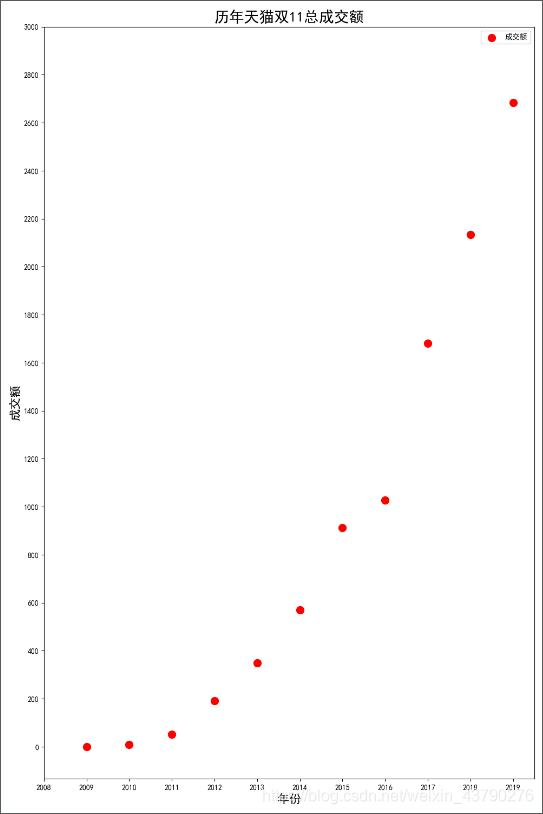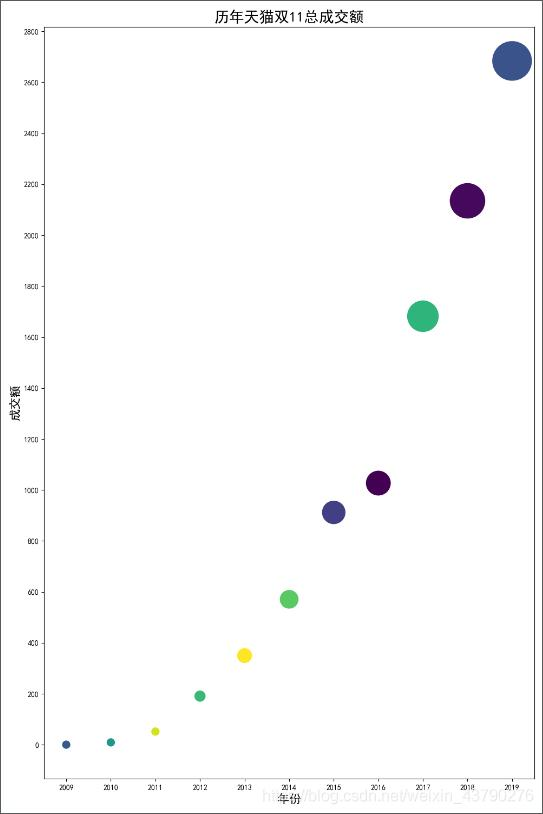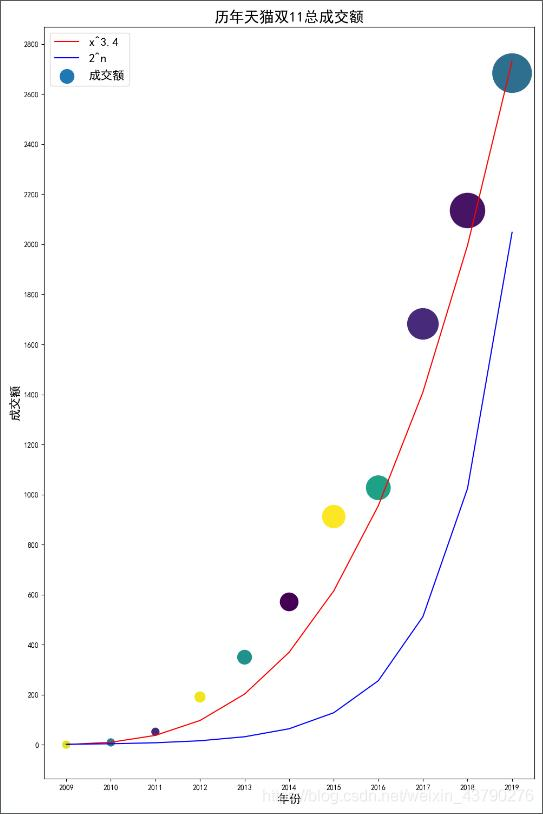# python怎么用散点图_Python利用matplotlib绘制散点图的新手教程

# coding=utf-8

import matplotlib.pyplot as plt

years = [2009, 2010, 2011, 2012, 2013, 2014, 2015, 2016, 2017, 2018, 2019]

turnovers = [0.5, 9.36, 52, 191, 350, 571, 912, 1027, 1682, 2135, 2684]

plt.figure(figsize=(10, 10), dpi=100)

plt.scatter(years, turnovers)

plt.show()scatter(): matplotlib中绘制散点图的函数。可以传入很多参数，一般传入两个列表，分别是散点图中的x值和y值。上面的例子中使用2009年至2019年这十一年天猫双11的总成交额数据。

import matplotlib.pyplot as plt

years = [2009, 2010, 2011, 2012, 2013, 2014, 2015, 2016, 2017, 2018, 2019]

turnovers = [0.5, 9.36, 52, 191, 350, 571, 912, 1027, 1682, 2135, 2684]

plt.figure(figsize=(10, 15), dpi=100)

plt.scatter(years, turnovers, c='red', s=100, label='成交额')

plt.xticks(range(2008, 2020, 1))

plt.yticks(range(0, 3200, 200))

plt.xlabel("年份", fontdict={'size': 16})

plt.ylabel("成交额", fontdict={'size': 16})

plt.title("历年天猫双11总成交额", fontdict={'size': 20})

plt.legend(loc='best')

plt.show()import matplotlib.pyplot as plt

import numpy as np

years = [2009, 2010, 2011, 2012, 2013, 2014, 2015, 2016, 2017, 2018, 2019]

turnovers = [0.5, 9.36, 52, 191, 350, 571, 912, 1027, 1682, 2135, 2684]

plt.figure(figsize=(10, 15), dpi=100)

size = list()

for tur in turnovers:

size.append(tur) if tur > 100 else size.append(100)

plt.xticks(range(2008, 2020, 1))

plt.yticks(range(0, 3200, 200))

plt.scatter(years, turnovers, c=np.random.randint(0, 50, 11), s=size)

plt.xlabel("年份", fontdict={'size': 16})

plt.ylabel("成交额", fontdict={'size': 16})

plt.title("历年天猫双11总成交额", fontdict={'size': 20})

plt.show()pip install numpy -i https://pypi.tuna.tsinghua.edu.cn/simple

import matplotlib.pyplot as plt

import numpy as np

import math

years = [2009, 2010, 2011, 2012, 2013, 2014, 2015, 2016, 2017, 2018, 2019]

turnovers = [0.5, 9.36, 52, 191, 350, 571, 912, 1027, 1682, 2135, 2684]

squares = [math.pow(year-2008, 3.3) for year in years]

powers = [math.pow(2, year-2008) for year in years]

plt.figure(figsize=(10, 15), dpi=100)

size = list()

for tur in turnovers:

size.append(tur) if tur > 100 else size.append(100)

plt.xticks(range(2008, 2020, 1))

plt.yticks(range(0, 3200, 200))

plt.scatter(years, turnovers, c=np.random.randint(0, 50, 11), s=size, label='成交额')

plt.plot(years, squares, color='red', label='x^3.4')

plt.plot(years, powers, color='blue', label='2^n')

plt.legend(loc='best', fontsize=16, markerscale=0.5)

plt.xlabel("年份", fontdict={'size': 16})

plt.ylabel("成交额", fontdict={'size': 16})

plt.title("历年天猫双11总成交额", fontdict={'size': 20})

plt.show()08-1423606-214208
01-144935
02-10320
07-139万+
12-152654
01-29580
08-05977
12-03957
10-282078
©️2020 CSDN 皮肤主题: 1024 设计师:白松林